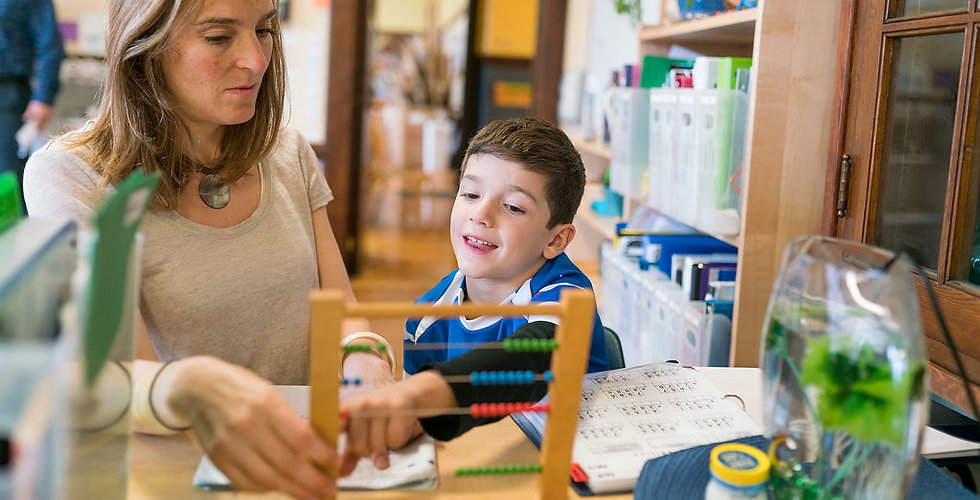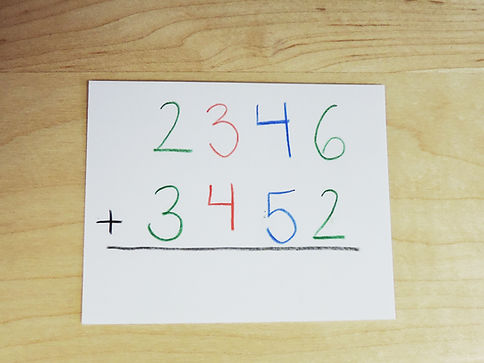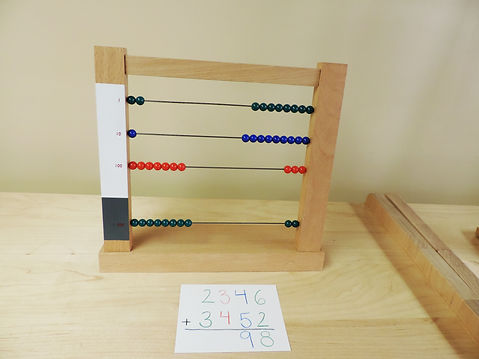##### FEATURED LESSON:

Curricular Area

Math

Level

Introduced to students beginning Lower Elementary, as ready

Skills#### The Small Bead Frame is a material students use to add, subtract, and multiply into the thousands.

Designed for children beginning Lower Elementary, the material helps children to move toward an abstract understanding of mathematical operations.

#### Solve the following equation: 2346 + 3452.#### 1 (units), 10 (tens), 100 (hundreds), and 1000 (thousands).

The color coding for units, tens, and hundreds is consistent across the majority Montessori materials.#### Make “2346” using the bead frame. Slide 6 units, 4 tens, 3 hundreds, and 2 thousands to the right side.#### Now add the units. In the number “3452” there are 2 units.#### How many units are there now? Count the beads and write down the answer: 8 units.#### Repeat this process for the tens column: 4 + 5 = 9.#### In the hundreds column: 3 + 4 = 7.#### In the thousands column: 2 + 3 = 5.